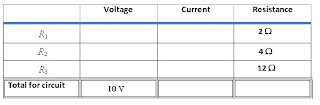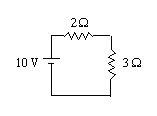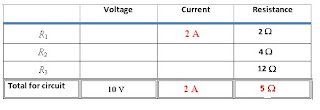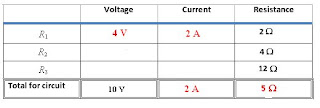Buy that special someone an AP Physics prep book, now with 180 five-minute quizzes aligned with the exam: 5 Steps to a 5 AP Physics 1

Visit Burrito Girl's handmade ceramics shop, The Muddy Rabbit: Yarn bowls, tea sets, dinner ware...

## 15 March 2009

### VIR charts and basic circuits

I got an email from Geoff Clarion the other day regarding VIR charts. Geoff graded AP physics exams with me for several years. He was known as the “cruise director” at the AP reading, because he didn’t just talk about how fun it would be to do something active and interesting – he actually made arrangements such that events happened. Geoff organized a yearly softball game*, volleyball nights, nights on the town, and so on.

I always say that the AP reading has been the best professional development I’ve ever been a part of. Many of my little tricks of the trade have been based on a snapshot of conversation from the reading. I mean, there I’m in the presence of 150 strong physics teachers, nerds like me who tend to talk shop when they’re not actually grading AP exams. I think it was Geoff who first suggested the utility of the green laser pointer as a demonstration tool. In turn, Geoff likes the VIR charts I use in my own class.

What is a VIR chart, you ask? It’s a straightforward way of organizing the answers to a circuit question, one which comes with a built-in reminder of when Ohm’s law is valid.

Everyone who takes physics can spit back Ohm’s law… V = IR. Wonderful. Problem is, too many students use this equation indiscriminately. Consider the basic circuit shown below. The goal is to determine the current through the 4 Ω resistor.“Ah,” says the novice, “the voltage is 10 V, the resistance is 4 Ω, so by ohm’s law I divide to get a current of 2.5 A.”

“Boux,” says the teacher.** Ohm’s law cannot be used as a bludgeon. To teach the proper use of Ohm’s law, I organize my solution using a VIR chart like the one here.The key to this chart: Ohm's law can ONLY be used when two of the three entries in a single row are known. Any other use of V=IR constitutes fraud.

Initially, I've only input the given values: the voltage of the battery, and the resistance of each individual resistor. Note that I know only one value in each row -- that indicates that there's more work to be done before Ohm's law is useful. The obvious approach is to find the equivalent resistance of the whole circuit. First I combine the parallel resistors to get this circuit:Now, it's clear that the total resistance must be 5 Ω. I plug that into the VIR chart:Voila, I have two of three entries in the "total" row completed. Ohm's law can be used to finish the row: I = V/R, giving a total current in the circuit of 2 A. Woo-hoo! A correct use of the law.

Warning: The VIR chart is not a magic square. It is not permissible just to add the resistances algebraically to get the total; we'll see that 2 A is NOT the sum of the "current" column. The chart is merely a method of organizing one's solution. It is only useful in conjunction with circuit diagrams.

With that reminder, I'll see what else I can figure out by looking at the circuit diagrams. By definintion, the "total" current in the circuit is the current coming out of the battery. Since R1 is in series with the battery, it must take the full current from the battery -- all 2 A. I put that in the chart:And now I have two entries in the top row, so I use Ohm's law to find the voltage across the first resistor:Almost done. Now the trick is to remember the rules about voltage in series and parallel resistors: The voltage across parallel resistors is the same, but the voltage across series resistors adds to the total. (That's just a simple statement of Kirchoff's loop rule.) Since the 2 Ω resistor takes 4 V, and the total is 10 V, the 3 Ω equivalent resistance must take the remaining 6 V. And, since voltage across parallel resistors is the same for each, I can fill in 6 V for BOTH R2 and R3. With two of the three entries in the rows, I can use Ohm's Law to find the missing currents.Checking the reasonability of my chart, I see that Kirchoff's junction rule is satisfied. The two parallel resistors take different currents that add to the total, and the smaller resistor takes more of the current. My work here is finished.
GCJ

* My stats: 2-4 with 2 runs scored, 3 assists and an error. Game MVP: Nebraska alumna Shelly Strand, my table leader at the time, whose defense at first base simply shut down the opposition (and saved me several more errors).

** “Boux” has sort of become a Jacobs catch phrase, as this is what I write on papers that show crazy mistakes like the one I’m describing here. The source of the phrase is an old Dave Barry column in which he asserts that Mets fans say, “Boo! You stupid bum!” while the more civilized Francophone Expos fans say, “Boux! Voux dumme bumme!” I’m paraphrasing, but that was the gist.

1.how did you find the resistance of the 2 parallel to be 3?

2.Use the formula for adding parallel resistors -- 1/R1 + 1/R2 = 1/R(equivalent). In this case, take 1/(4 ohms) plus 1/(12 ohms), then reciprocate the answer to get an equivalent resistance of 3 ohms.

GCJ

3.how did you get 5 as the total of the RESISTOR??

4.Look at the diagram where the 2 ohm resistor and the 3 ohm resistor are in series. Add up the series resistances to get 5 ohms.

5.how can you get 1/4 +1/12=3

6.Add 1/4 + 1/12 to get 4/12... then take the recipricol of this answer to get 12/4 = 3. The equivalent resistance is 3 ohms.

7.I use your chart too - tho I added Power - so it's a VIRP chart - which allows for sooooo many good puns! It also lets me use power to explain why some bulbs end up dimmer than others...keep it coming! I never fail to pick up a few new tricks every read of your blog!

8.I'm curious about the green laser pointer? I have one diffraction labs, what is this useful demonstration you speak of?